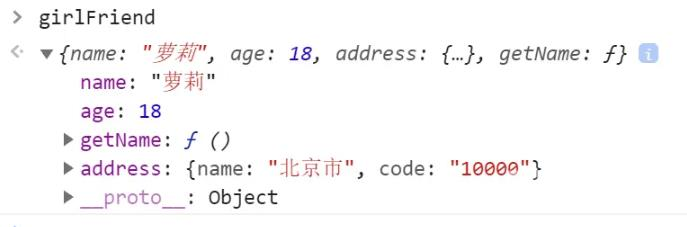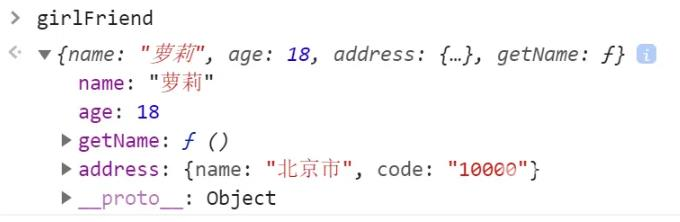﻿ JavaScript对象的四种创建方法 - 自由资讯

# JavaScript对象的四种创建方法

##### 目录
• 前言
• 1. 基于对象字面量
• 2. 利用new Object方式创建对象
• 3. 基于构造函数
• 4. 基于工厂方法

## 1. 基于对象字面量

``` /* 1.基于对象字面量 */
let girlFriend = {
name: "萝莉",
age: 18,
getName: function() {
return this.name
},
name: '北京市',
code: '10000'
}
}```

## 2. 利用new Object方式创建对象

``` // 2. 利用new Object方式创建对象
this.name = name
this.age = age
this.setName = function() {
return (this.name)
}
}
//利用new 来创建对象
var girlFriend1 = new GirlFriend("萝莉", 18, {
name: '北京市',
code: '10000'
})```## 3. 基于构造函数

``` // 3. 基于构造函数
this.name = name
this.age = age
this.setName = function() {
return (this.name)
}
}
//利用new 来创建对象
var girlFriend1 = new GirlFriend("萝莉", 18, {
name: '北京市',
code: '10000'
})```

## 4. 基于工厂方法

``` // 4.基于工厂方法
let o = new Object();
o.name = name;
o.age = age;
o.setName = function() {
return this.name
}
return o;
}
let girlFriend = createGirlFriend('萝莉', 18, {
name: '北京市',
code: '10000'
})```﻿